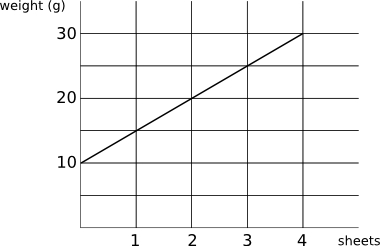The graph shows how the weight of a letter (including the envelope) varies with the number of sheets of paper used.

What is the weight of a single sheet of paper?

What is the weight of the envelope?

If w is the weight of the letter (including the envelope) and s is the number of sheets of paper, what is equation giving w as a function of s (the equation of the line)?